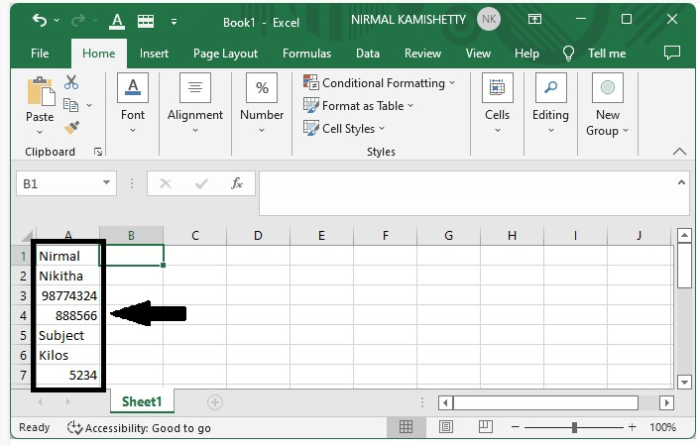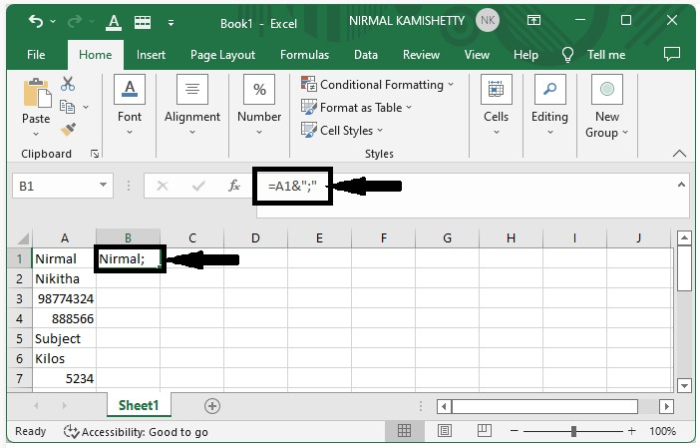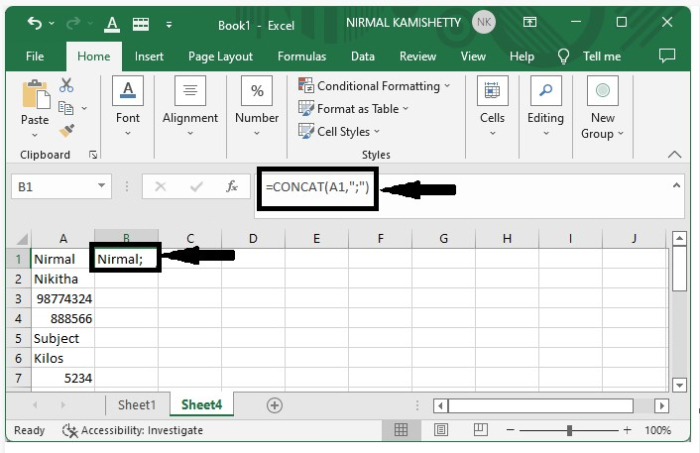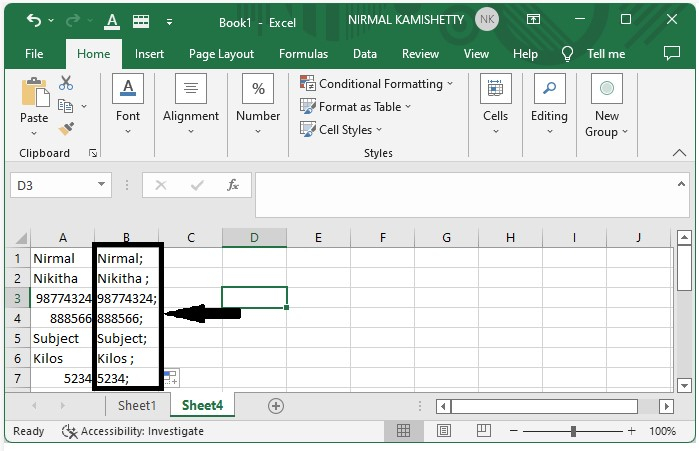# How to Add Semicolon to Each Cell in Excel?

If you have a huge Excel sheet with lots of data and there is a requirement to add a semicolon to each cell, then you wouldn't surely do it manually. In this tutorial, we will show you a simple hack that you can use for this purpose.

## Adding Semicolon to Each Cell Using "&" Operator

Let's start with the first method. You can add a semicolon to each cell using the "&" operator.

### Step 1

Let us consider we have the following Excel sheet that contains a list of numbers and names. We will be using the concept of adding suffix to a list of data.Click an empty cell where you want to show the first result and then enter the formula =A1&";" in the formula box and hit the "Enter" button. It will append a semicolon to the text given in "A1". See the following screenshot:### Step 2

To get all the other results, just darg down from the corner till all the results are filled.## Adding Semicolon to Each Cell using CONCAT

This is the second method. You can use the CONCAT method to concatenate a semicolon at the end of each cell.

### Step 1

Let us consider the same data which we used in the above example. Click an empty cell where you want to display the first result, enter the formula =concat(A1,";") in the formula box, and hit the "Enter" button. It will produce the following result:### Step 2

To get all the other results, just darg down from the corner till all the results are filled. Our final output will look like the one shown below:## Conclusion

In this tutorial, we used two different methods to demonstrate how you can add a semicolon to the existing data in an Excel sheet in a very easy and efficient way.